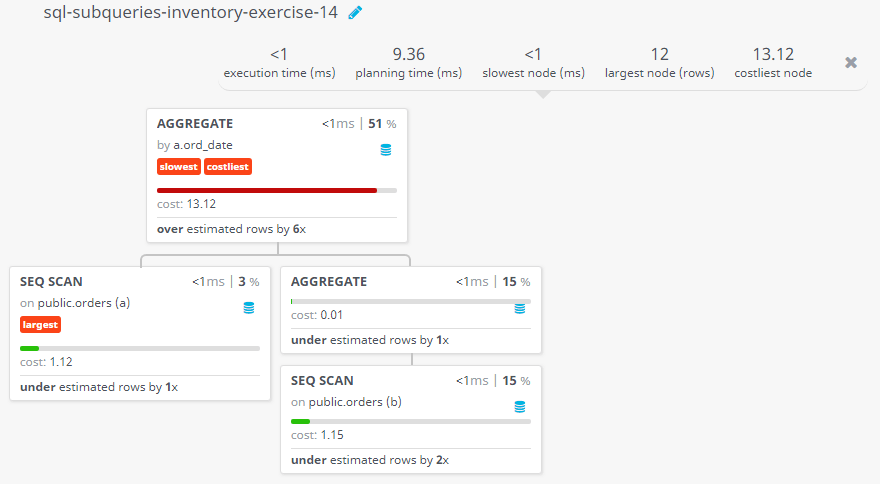﻿ SQL SUBQUERIES: Find the sums of the order amounts, grouped by date, eliminating all those dates where the sum was not at least 1000.00 above the MAX amount for that date - w3resource

# SQL Subquery Exercises: Find the sums of the order amounts, grouped by date, eliminating all those dates where the sum was not at least 1000.00 above the MAX amount for that date

## SQL SUBQUERY: Exercise-14 with Solution

14. Write a query to find the sums of the amounts from the orders table, grouped by date, eliminating all those dates where the sum was not at least 1000.00 above the maximum amount for that date.

Sample table: Orders

Sample table: Customer

Sample Solution:

``````SELECT ord_date, SUM (purch_amt)
FROM orders a
GROUP BY ord_date
HAVING SUM (purch_amt) >
(SELECT 1000.00 + MAX(purch_amt)
FROM orders b
WHERE a.ord_date = b.ord_date);
``````

Output of the Query:

```ord_date	sum
2012-09-10	6979.15
2012-10-10	4463.83
```

Explanation: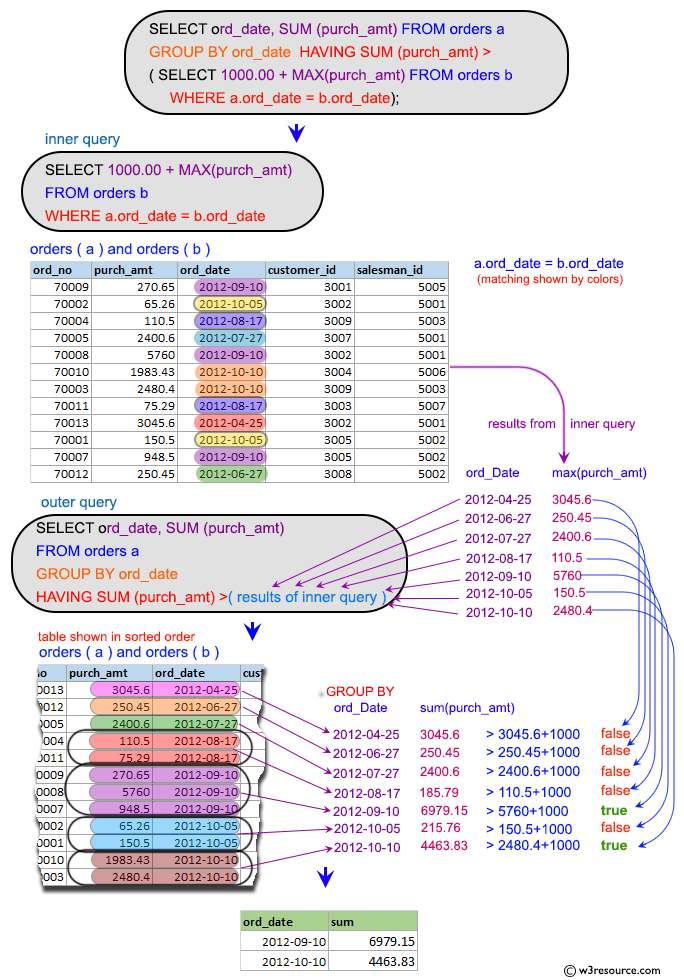## Practice Online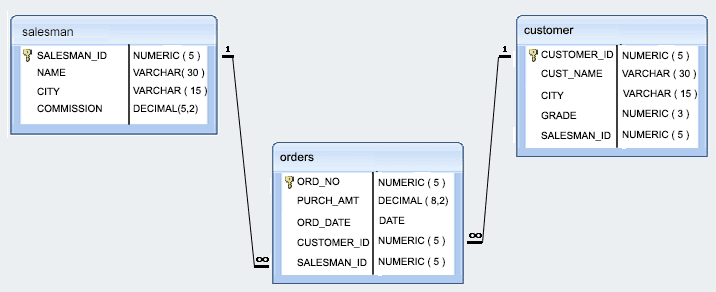## Query Visualization:

Duration: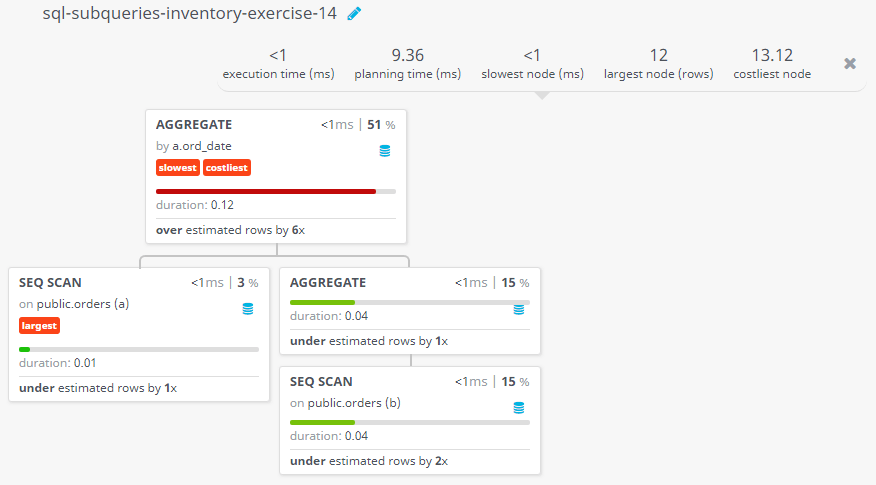Rows: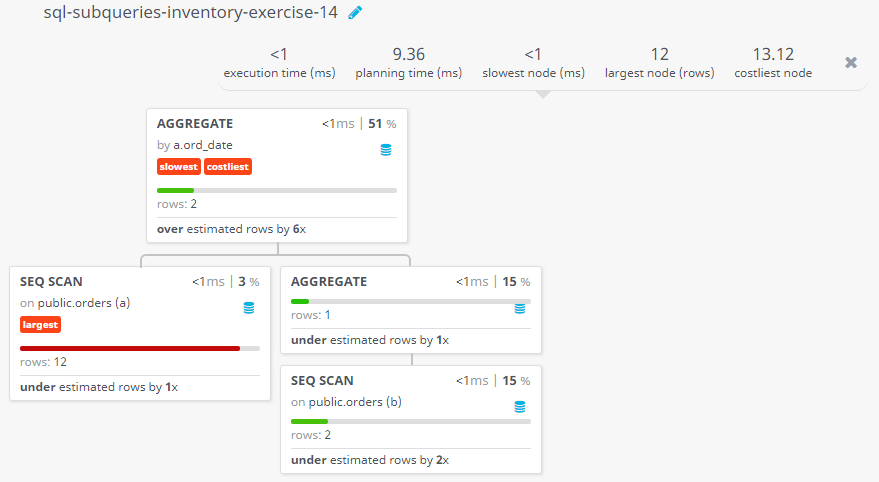Cost: